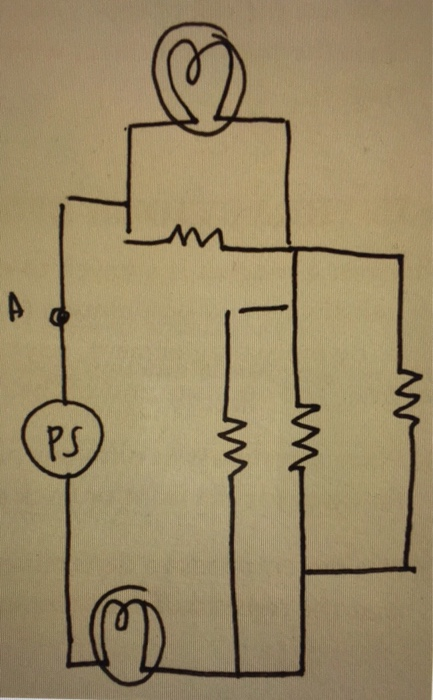# Problem: For this diagram, include three extra circuit symbols:A. An ammeter measuring the current at point AB. A voltmeter measuring the voltage drop across the topmost resistor (the one in parallel with the topmost bulb)C. An ammeter measuring the current through the rightmost resistor

###### FREE Expert Solution

Note:

An ammeter is connected in series with circuit elements.

A voltmeter is connected in parallel with a circuit element.

99% (143 ratings)###### Problem Details

For this diagram, include three extra circuit symbols:

A. An ammeter measuring the current at point A

B. A voltmeter measuring the voltage drop across the topmost resistor (the one in parallel with the topmost bulb)

C. An ammeter measuring the current through the rightmost resistor